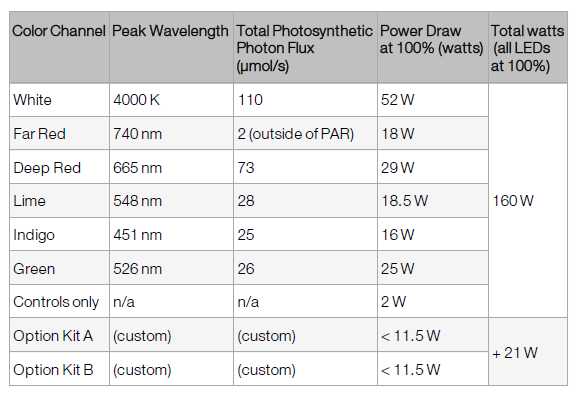# How to calculate power consumption for a specific spectrum / for a whole Alina System

## Description/Explanation of Issue

How much power is my Alina Luminaires of system using?

## Fix/Solution

1. In the Alina User Manual there is a table of the power consumption for the Alina fixture head: (See link Below)2.   So an Alina head "everything at full blast" would be add up to 181W.

3. So If you run a specific spectrum, you can pro-rate the above full power level per color and calculate the resulting power consumption.  Example: If you were running only the White and Far Red the output would add up to 70W.

4. Take this number and add ~10% for the power supply. Example: 181 + 10% = 199W

5. Total power used, times the number of fixtures connected to the power supply Example: 8 Fitures * 199W = 1592W

6. To get power consumption on electrical circuit, Divide the total watts by the voltage (110v or 240v).

Example 1 : 1592W / 110 = 14.5Amps per Circuit.    Example 2 : 1592W / 240 = 6.6Amps per Circuit.     Current draw would be less that this if not everything is at full.